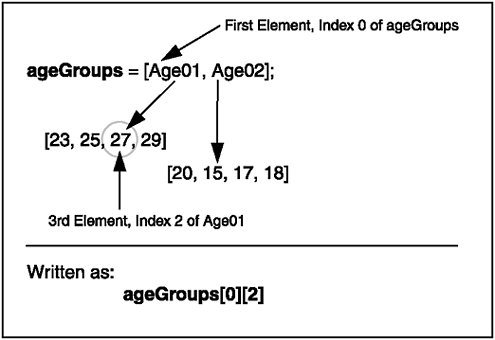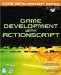# Multi-Dimensional Arrays

 [ LiB ]

In the past sections, you've been dealing with one-dimensional arrays. They are useful for many tasks like storing the last 10 locations of your mouse cursorthis would be useful when creating a mouse trail. In this section, you will explore multi-dimensional arrays . These are special arrays that contain other arrays within one or more of their elements. The number of arrays embedded determines how many dimensions the array is considered to be. Two-dimensional arrays can also be used as matrixes , depending on how you look at it.

One use for multi-dimensional arrays is in a situation where you need to store different sets of data into different elements of the array. An example would be an array of spaceship enemies in a game. You can store individual sets of information (of each enemy ship) as an array, and then take all these sets and store them in one bigger array for even easier accessthis would then include all the enemies in space. Once you have this multi-dimensional array setup, you'll be able to access all the properties of all the enemies in the world just by using one alias.

NOTE

NOTE

There are many uses for multi dimensional arrays, but for the demonstration purposes in this sec tion I will only discuss two-dimen sional arrays.This will help you bet ter understand the concepts quicker and more clearly.

So how would you declare a 2D array? There are a couple of ways to do so. Here's one way:

` Age01 = [23, 25, 27, 29]; Age02 = [20, 15, 17, 18]; ageGroups = [Age01, Age02]; `

This example shows the creation of three arrays. The first two have four elements each. These arrays are used as initialization values when creating ageGroups . What this means is that you can now access all those values through one alias, ageGroups . Take a look at the following syntactic example:

` mainArray[whichElement][whichElementInSubAlias] `

mainArray would be ageGroups , in this case. The first pair of brackets indexes the element you want to further reference. In other words, by stating mainArray , internally, you are indexing Age02 because it is the second element in ageGroups. The second pair of brackets indexes whatever element is in Age02 .

Just to give you an example, ageGroups will yield the value 27. Why? The 0 index is referencing Age01 , and within Age01 , the 2 index is referencing the third element. Check out Figure 5.3 before moving on.

##### Figure 5.3. Indexing a 2D arrayIf you understand what we have gone over so far, you should easily understand how more arrays could be embedded into other arrays to create complex structures.

Let's look at other ways that you could declare multi-dimensional arrays. The following example shows you how to declare multi-dimensional arrays with the array constructor:

` multiArray = [50, new Array(50)]; `

Don't let yourself be fooled by the last statement. Look at it closely and then decide what it really does. multiArray is a 2x50 2-D array. The first element in it is the value 50 and index 1 is another array of another 50 elements (embedded within multiArray ).

Of course, as you can see from our example, the 50 elements embedded in the second element are not initialized . You can put this array in a loop, such as the one in my following example, to loop through each element for initialization:

` for (var index = 0; index < 50; index++) {   // The value of index is being assigned   // to the index'th element in multiArray.   multiArray[index] = index; } `

Looks a little complex, but it's not. You already know how to loop for 50 iterations, but as a refresher, let's go through it anyway. The for loop initializes the local index variable to 0. It then loops while index is less than 50. After each iteration, the for loop increases index by 1.

If you notice carefully , multiArray's second index is the index relative to the loop's indexing variable during each iteration. What in the world does that mean? It means that when the index variable is 0, multiArray will be indexing like this: multiArray . When index is 1, it will be indexing like this: multiArray , and so on.

At the same time, during each iteration, index is the value being assigned. That means that if you were to list the 50 elements (embedded in the second element of multiArray) , you would get a list of numbers from 0 to 49. Why? Because index starts at 0 and ends at 49 because the loop becomes false and exits at that time.

NOTE

TIP

If you are having trouble visualizing this loop, I suggest breaking it down into smaller pieces. First thing you should do is add a trace command to the block of code to verify what is being put into the array during each iteration of the loop. Also try assign ing a different value instead of assigning index . These are just a few tips to help you understand code. It is also a good idea to comment your code.

 [ LiB ]Game Development with ActionScript
ISBN: 1592001106
EAN: 2147483647
Year: 2004
Pages: 162
Authors: Lewis Moronta